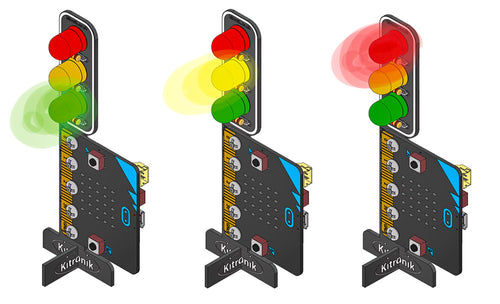Unlock your students potential with these 3 free Lesson Plans for our STOP:bit for the BBC microbit. Students will learn how to code traffic lights and pedestrian crossings with these classroom ready teaching resources.They have been designed to introduce Key Stage 3 students to embedded systems. All three lessons include curriculum mapping and also practical challenges for KS3 students. We've gone into as much detail as possible so you can get these into the classroom with as little fuss as possible.

## Teachers Lesson Plans For STOP:bit For microbit:

Firstly, the ZIP file for these lesson plans contains three PDFs, one for each lesson.

### Lesson 1 - Traffic Light Sequence:This is an introductory lesson for Key Stage 3 students to embedded systems. The lesson involves the teacher discussing how we make traffic lights coordinate traffic. First in plain English, then through algorithms, then through code. The teacher will write the algorithms on the board and the students will then create the code using the algorithm as a guide. The recommended ratio of students to STOP:bits is 2:1.

### Lesson 2 - Pedestrian Crossing:This is an introductory lesson for Key Stage 3 students to embedded systems. The lesson involves the teacher discussing how we interact with traffic lights to enable pedestrian crossings. First in plain English, then through algorithms, then through code. The teacher will write the algorithms on the board and the students will then create the code using the algorithm as a guide. The recommended ratio of students to STOP:bits is 2:1.

### Lesson 3 - Traffic Light & Pedestrian Crossing:This is a follow-up lesson for Key Stage 3 students on embedded systems. The lesson involves the teacher discussing how we make traffic lights that also coordinate traffic with pedestrians. First in plain English, then through algorithms, then through code. The teacher will write the algorithms on the board and the students will then create the code using the algorithm as a guide. The recommended ratio of students to STOP:bits is 2:1.
Finally, the ZIP file for these lesson plans contains three PDFs, one for each lesson.